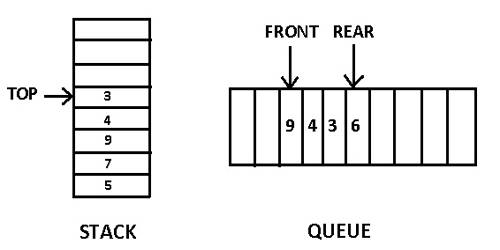Technology

# Basic difference between Stack and QueueStack and Queue both are the non-primitive data structures. A stack is one ended whereas the queue is double ended. The main difference is Stack follows LIFO mechanism on the other hand Queue follows FIFO mechanism to add and remove elements.

The differences between stack and queue are given below:

Stack – It is a linear data structure used to organize data in a particular way so that it can be used efficiently.

• Stack insertion and deletion operations are performed the same end.
• In the stack, an element which inserted last is first to delete, so at it called last in first out.
• In the stack, only one pointer is used called TOP.
• In the stack, there is no space of memory space.
• Plate counter at Marriage reception is an example of a stack.
• Stack uses LIFO (last in first out) method to access.
• A Stack requires only one reference pointer.
• A Stack cannot be divided into subsections and it doesn’t have extensions.

Queue – It is also a linear data structure, somewhat similar to a stack data structure, except it is open at both the ends.

• In queue insertion and deletion operation are performed at operation are performed different.
• In queue an element which inserted first in first out to delete. So it is called first in first out.
• In QUEUE two pointers are used ailed FRONT and REAR.
• In queue, there is wastage of memory space.
• Students standing in a line also counted are an example of a queue.
• Queue uses FIFO (First in first out) method to access and add data elements.
• A Queue requires two reference pointers.
• A Queue can be divided into subsections and it has the following extensions: Double Ended Queue, Simple Queue, Priority Queue and Circle Queue.